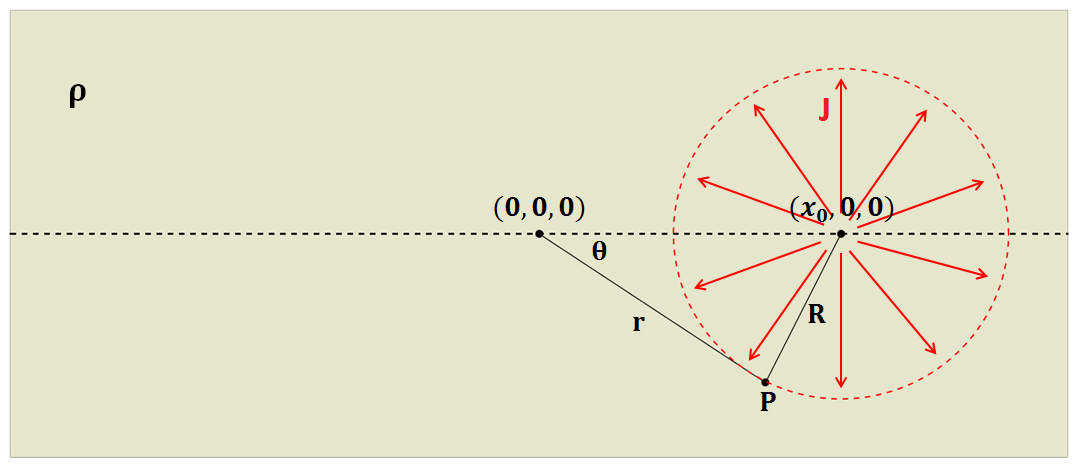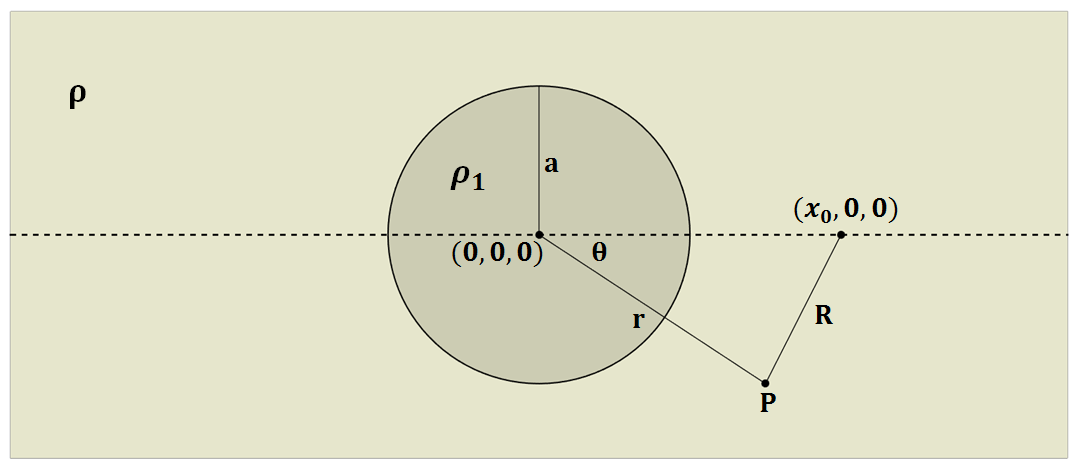# Point current source and a conducting sphere

In this section, we build up an analytical solution for a conducting sphere in the presence of a point current source. This is accomplished in two steps:

1. A solution for the electric scalar potential, due to a point current source within a wholespace of resistivity $$\rho$$, is solved. This solution is then re-expressed within a polar coordinate system and decomposed into a sum of spherical harmonic modes.

2. The solution for a conducting sphere within a wholespace is then determined by solving a boundary value problem for each spherical harmonic.

This solution is built upon in Effects of Topography to investigate the effects of of a hemispherical depression at the Earth’s surface on the behaviour of currents and potentials in a direct current resistivity experiment.

## Electric Potential from a Current Source within a Wholespace

Let us consider the case where an electrical current $$I$$ is being injected into a wholespace with resistivity $$\rho$$, at location ($$x,y,z$$) = ($$x_0,0,0$$). Assuming the medium is lossless, this results in a current density $$J$$ which flows radially outwards from the source, with magnitude:

$J = \frac{I}{4 \pi R^2}$Fig. 80 Diagram showing the setup for computing the potential due to a wholespace.

where $$R$$ is the distance from the source to a point of measure $$P$$, and $$4\pi R^2$$ is the area of a ball centered at the source. Because our problem is electrostatic, $$\vec E = - \nabla \phi$$ according to Faraday’s law. The scalar electric potential $$\phi$$ can be obtained by integrating the electric field from $$R$$ to $$\infty$$. By substituting Ohm’s law ($$\vec E = \rho \vec J$$) into the path integral:

$\phi = - \int_R^\infty \vec E \cdot d\vec l = - \int_R^\infty \frac{\rho I}{4 \pi r^2} dr = \frac{\rho I}{4\pi R}$

For reasons which will becomes apparent in the next section, we would like to re-express $$\phi$$ in terms of a radial coordinate system ($$r,\theta,\phi$$), centered at ($$x,y,z$$) = (0,0,0). Because the points which represent the problem geometry do not necessarily form a right-triangle, $$R$$ must be expressed using the cosine law:

$R = \sqrt{x_0^2 + r^2 - 2rx_0 cos \theta \;}$

For solutions where $$r<x_0$$, $$1/R$$ can be split into a sum of spherical harmonic modes using the binomial theorem:

(354)$\begin{split}\frac{1}{R} &= \frac{1}{x_0} \Bigg [ 1 + \Bigg ( \frac{r}{x_0} \Bigg )^2 - 2 \frac{r}{x_0} cos \theta \Bigg ]^{-1/2} \\ &= \frac{1}{x_0} \Bigg [ 1 + \frac{r}{x_0}cos \theta + \Bigg ( \frac{r}{x_0} \Bigg )^2 \Bigg ( \frac{3}{2} cos^2 \theta - \frac{1}{2} \Bigg ) + \; \dotsb \; \Bigg ] \\ &= \frac{1}{x_0} \sum_{n=0}^\infty \Bigg ( \frac{r}{x_0} \Bigg )^n P_n \big (cos \theta \big)\end{split}$

where $$P_n \big (cos \theta \big )$$ is the Legendre polynomial of order $$n$$. Because Legendre polynomials have magnitudes less than unity for $$n>0$$, and $$r<x_0$$, the infinite series in Eq. (354) is bounded and converges as $$n \rightarrow \infty$$. A similar approach for $$r > x_0$$ can be expressed as follows:

(355)$\begin{split}\frac{1}{R} &= \frac{1}{r} \Bigg [ 1 + \Bigg ( \frac{x_0}{r} \Bigg )^2 - 2 \frac{x_0}{r} cos \theta \Bigg ]^{-1/2} \\ &= \frac{1}{r} \Bigg [ 1 + \frac{x_0}{r}cos \theta + \Bigg ( \frac{x_0}{r} \Bigg )^2 \Bigg ( \frac{3}{2} cos^2 \theta - \frac{1}{2} \Bigg ) + \; \dotsb \; \Bigg ] \\ &= \frac{1}{r} \sum_{n=0}^\infty \Bigg ( \frac{x_0}{r} \Bigg )^n P_n \big ( cos \theta \big )\end{split}$

Similar to Eq. (354), since $$x_0<r$$, the infinite series in Eq. (355) is also boundary and conveges as $$n\rightarrow\infty$$. Therefore using Eqs. (354) and (355), the electric scalar potential $$\phi$$ can be expressed as an infinite sum of spherical harmonic modes, where:

(356)$\phi = \frac{I\rho}{4 \pi} \sum_{n=0}^\infty \frac{r^n \, P_n \big (cos \theta \big )}{x_0^{n+1}} \; \; \; \textrm{for} \; \; \; r<x_0$

and

(357)$\phi = \frac{I\rho}{4 \pi} \sum_{n=0}^\infty \frac{x_0^n \, P_n \big (cos \theta \big )}{r^{n+1}} \; \; \; \textrm{for} \; \; \; x_0<r$

Unfortunately, this method cannot be used to find a bounded and convergent series for $$r=x_0$$.

## Electric Potential for a Conducting Sphere in a Wholespace

Let us now consider the electrical scalar potential at $$P$$ in the presence of a conducting sphere of radius $$a$$ and resistivity $$\rho_1$$, centered at the origin. Once again, a current of $$I$$ is injected at ($$x_0,0,0$$). Due to the radial symmetry of the problem, $$\partial /\partial \phi = 0$$. Away from the source, the electric field is divergence free. As a result, $$\phi$$ can be expressed in terms of the following 2d Poisson’s equation:

(358)$\nabla^2 \phi = \frac{1}{r} \frac{\partial }{\partial r} \big ( r^2 \big ) \frac{\partial \phi}{\partial r} + \frac{1}{r^2 sin \theta} \frac{\partial}{\partial \theta} \Bigg ( sin \theta \frac{\partial \phi}{\partial \theta} \Bigg ) = 0$

The boundary conditions for our problem state that $$\phi$$, and current flow normal to the sphere’s surface, are continuous at $$r=a$$. Therefore:

(359)$\phi = \phi_1 \; \; \; \textrm{and} \; \; \; \frac{1}{\rho} \frac{\partial \phi}{\partial r} = \frac{1}{\rho_1} \frac{\partial \phi_1}{\partial r} \; \; \; \textrm{at} \; \; \; r=a$

For a source which is outside the sphere ($$a < x_0$$), the desired solution for the potential is:

(360)$\phi = \frac{I \rho}{4\pi R} + \sum_{n=0}^\infty A_n \frac{1}{r^{n+1}} P_n \big ( cos \theta \big ) \; \; \; \textrm{for} \; \; \; r>a$

and

(361)$\phi_1 = \sum_{n=0}^\infty B_n r^n P_n \big ( cos \theta \big ) \; \; \; \textrm{for} \; \; \; r<a$

This makes sense considering $$1/r$$ terms within the sphere would be infinite as $$r \rightarrow 0$$, and $$r$$ terms outside the sphere would be infinite as $$r \rightarrow \infty$$. Because Legendre polynomials can be used to form an orthogonal set of basis functions, coefficients $$A_n$$ and $$B_n$$ may be determined independently for each $$n$$. Using locations $$r<x_0$$, Eq. (356) can be substituted into Eq. (360). This can be use to solve Eq. (358), using boundary conditions from (359) for each harmonic mode $$n$$. The resulting coefficients are given by:

$A_n = \frac{I \rho}{4\pi} \Bigg ( \frac{a^{2n+1}}{x_0^{n+1}} \Bigg ) \Bigg ( \frac{n \big ( \rho_1 - \rho \big )}{n\rho + \big (n+1 \big )\rho_1} \Bigg )$

and

$B_n = \frac{I\rho}{4\pi} \Bigg ( \frac{1}{x_0^{n+1}} \Bigg ) \Bigg ( \frac{\big ( 2n+1 \big )\rho_1}{n\rho + \big ( n+1 \big )\rho_1} \Bigg )$

Therefore, the electric scalar potential observed outside the sphere is equal to:

(362)$\phi (r, \theta ,\phi) = \frac{I\rho}{4 \pi} \Bigg [ \frac{1}{R} + \sum_{n=0}^\infty \frac{a^{2n+1}}{\big (x_0 \, r \big )^{n+1}} \Bigg ( \frac{n \big ( \rho_1 - \rho \big )}{n\rho + \big (n+1 \big )\rho_1} \Bigg ) P_n \big ( cos \theta \big ) \Bigg ]$

Eq. (362) can be split into two terms: the potential for a wholespace from Eq. (357), and an anomalous potential which results from the exstence of a conducting sphere. Python code functions which evaluate above solution is given at DC sphere code.Fig. 81 Diagram showing the setup for computing the potential due to a conductive sphere in a wholespace.

### Variables

 $$\rho$$ Resistivity of the whole-space $$\rho_1$$ Resistivity of the sphere $$(0,0,0)$$ Origin of the coordinate and center location of the sphere $$(\pm x_0,0,0)$$ Location of the point current source, which has to be alined with $$x$$-axis $$x_0$$ Distance from current source from the origin (a postive scalar value) $$r$$ Distance from the origin to the measurement point $$P(x,y,z)$$ $$R$$ Distance between the measurement point ($$P$$) and the point current source $$\theta$$ Angle between the measurement point ($$P$$) and the point current source $$a$$ Radius of the sphere (m) $$I$$ Intenisty of the current $$\phi$$ Total potential outside of the sphere ($$r > a$$) $$\phi_1$$ Total potential inside of the sphere ($$r < a$$)